# 8 2 Trigonometric Ratios Warm Up Write each

• Slides: 198 -2 Trigonometric Ratios Warm Up Write each fraction as a decimal rounded to the nearest hundredth. 1. 2. 0. 67 0. 29 Solve each equation. 3. Holt Geometry x = 7. 25 4. x = 7. 99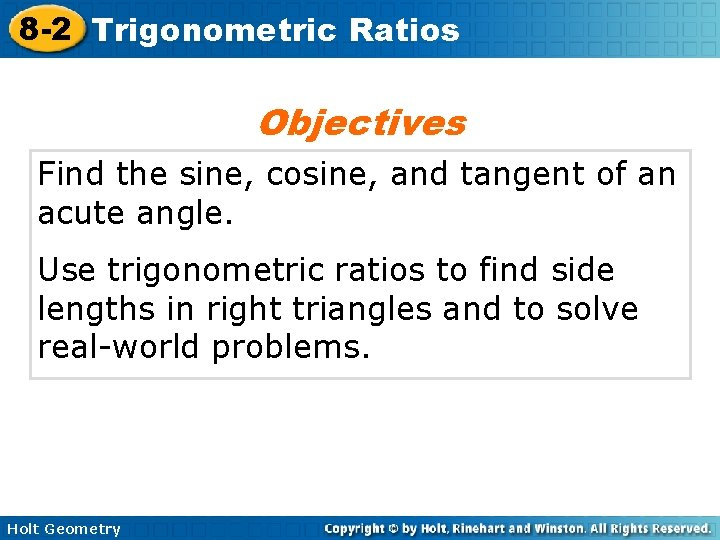8 -2 Trigonometric Ratios Objectives Find the sine, cosine, and tangent of an acute angle. Use trigonometric ratios to find side lengths in right triangles and to solve real-world problems. Holt Geometry8 -2 Trigonometric Ratios Holt Geometry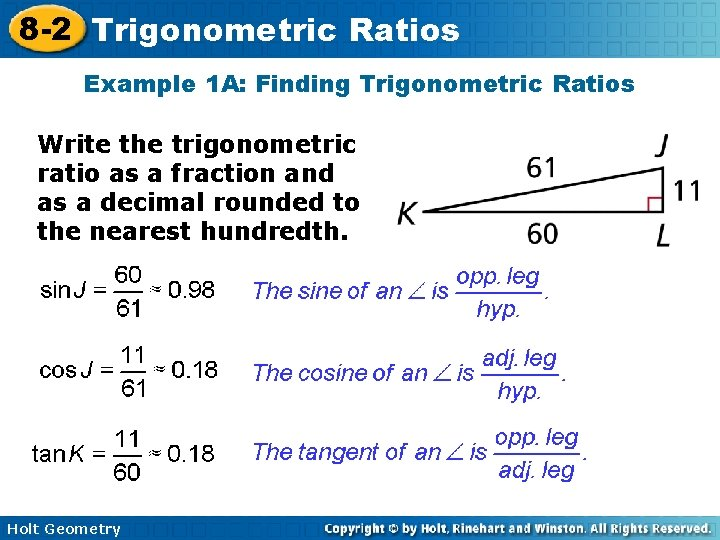8 -2 Trigonometric Ratios Example 1 A: Finding Trigonometric Ratios Write the trigonometric ratio as a fraction and as a decimal rounded to the nearest hundredth. Holt Geometry8 -2 Trigonometric Ratios Check It Out! Example 1 a Write the trigonometric ratio as a fraction and as a decimal rounded to the nearest hundredth. Holt Geometry8 -2 Trigonometric Ratios Example 2: Finding Trigonometric Ratios in Special Right Triangles Use a special right triangle to write cos 30° as a fraction. Draw and label a 30º-60º-90º ∆. Holt Geometry8 -2 Trigonometric Ratios Check It Out! Example 2 Use a special right triangle to write tan 45° as a fraction. Draw and label a 45º-90º ∆. 45° s Holt Geometry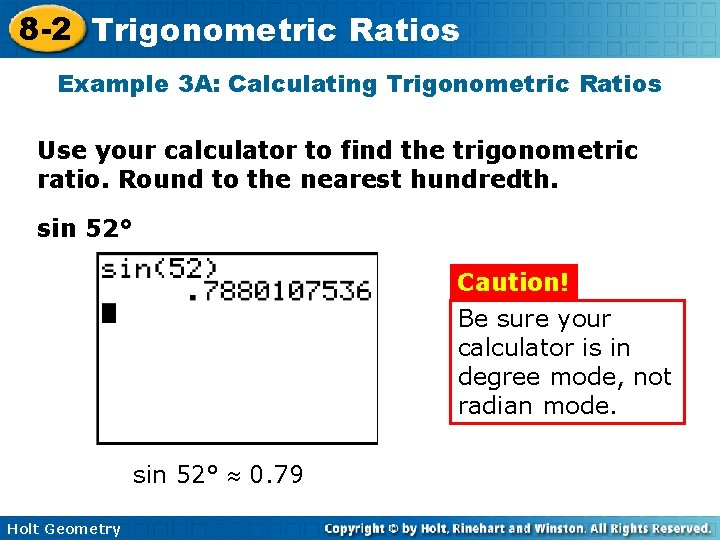8 -2 Trigonometric Ratios Example 3 A: Calculating Trigonometric Ratios Use your calculator to find the trigonometric ratio. Round to the nearest hundredth. sin 52° Caution! Be sure your calculator is in degree mode, not radian mode. sin 52° 0. 79 Holt Geometry8 -2 Trigonometric Ratios Example 3 B: Calculating Trigonometric Ratios Use your calculator to find the trigonometric ratio. Round to the nearest hundredth. cos 19° 0. 95 Holt Geometry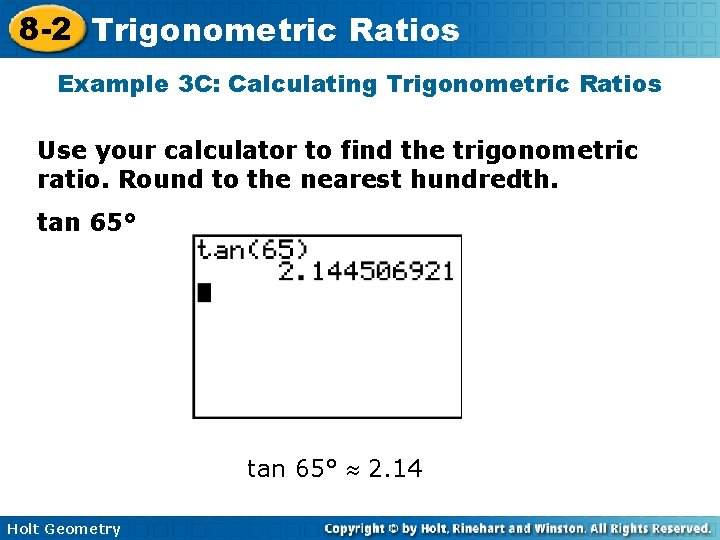8 -2 Trigonometric Ratios Example 3 C: Calculating Trigonometric Ratios Use your calculator to find the trigonometric ratio. Round to the nearest hundredth. tan 65° 2. 14 Holt Geometry8 -2 Trigonometric Ratios Example 4 A: Using Trigonometric Ratios to Find Lengths Find the length. Round to the nearest hundredth. BC is adjacent to the given angle, B. You are given AC, which is opposite B. Since the adjacent and opposite legs are involved, use a tangent ratio. Holt Geometry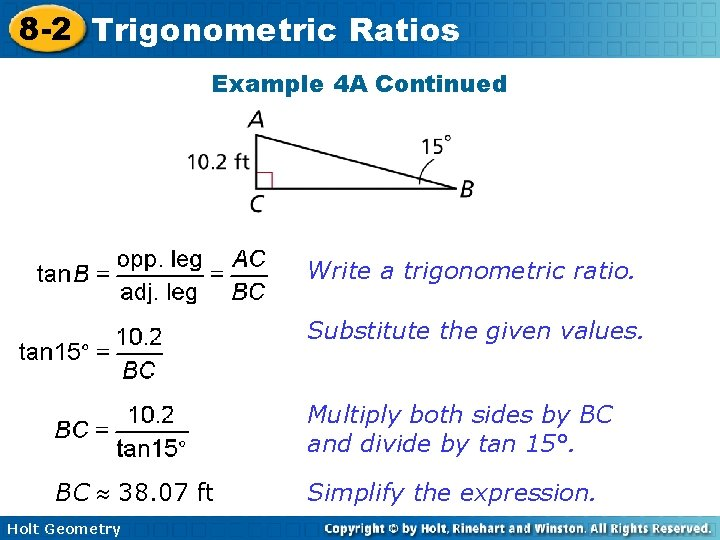8 -2 Trigonometric Ratios Example 4 A Continued Write a trigonometric ratio. Substitute the given values. Multiply both sides by BC and divide by tan 15°. BC 38. 07 ft Holt Geometry Simplify the expression.8 -2 Trigonometric Ratios Example 4 B: Using Trigonometric Ratios to Find Lengths Find the length. Round to the nearest hundredth. QR is opposite to the given angle, P. You are given PR, which is the hypotenuse. Since the opposite side and hypotenuse are involved, use a sine ratio. Holt Geometry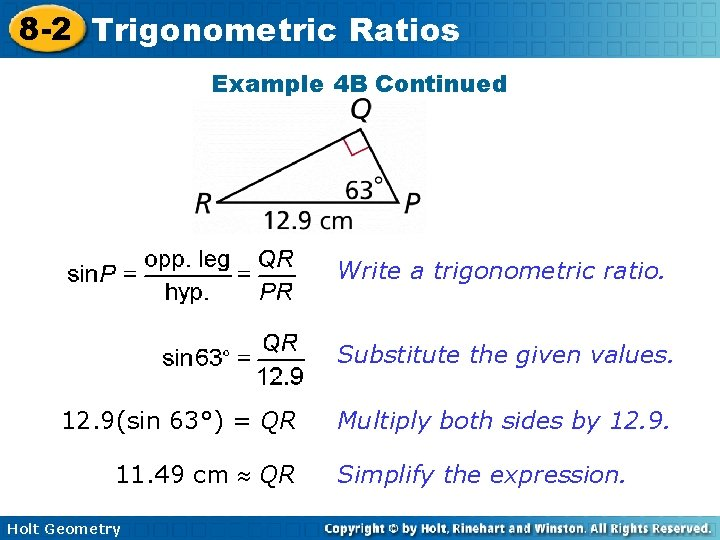8 -2 Trigonometric Ratios Example 4 B Continued Write a trigonometric ratio. Substitute the given values. 12. 9(sin 63°) = QR 11. 49 cm QR Holt Geometry Multiply both sides by 12. 9. Simplify the expression.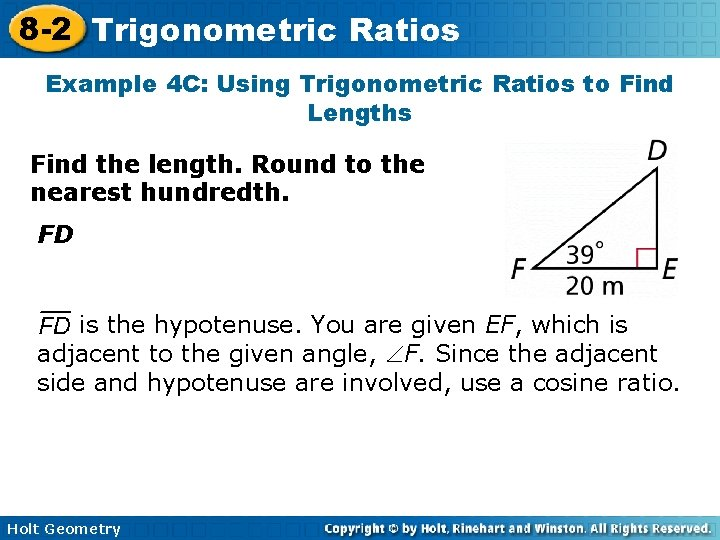8 -2 Trigonometric Ratios Example 4 C: Using Trigonometric Ratios to Find Lengths Find the length. Round to the nearest hundredth. FD is the hypotenuse. You are given EF, which is adjacent to the given angle, F. Since the adjacent side and hypotenuse are involved, use a cosine ratio. Holt Geometry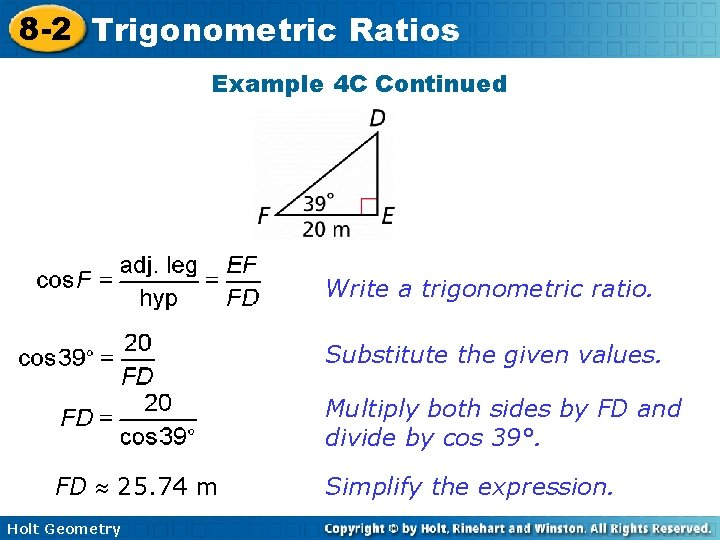8 -2 Trigonometric Ratios Example 4 C Continued Write a trigonometric ratio. Substitute the given values. Multiply both sides by FD and divide by cos 39°. FD 25. 74 m Holt Geometry Simplify the expression.8 -2 Trigonometric Ratios Lesson Quiz: Part I Use a special right triangle to write each trigonometric ratio as a fraction. 1. sin 60° 2. cos 45° Use your calculator to find each trigonometric ratio. Round to the nearest hundredth. 3. tan 84° 9. 51 Holt Geometry 4. cos 13° 0. 978 -2 Trigonometric Ratios Lesson Quiz: Part II Find each length. Round to the nearest tenth. 5. CB 6. AC 6. 1 16. 2 Use your answers from Items 5 and 6 to write each trigonometric ratio as a fraction and as a decimal rounded to the nearest hundredth. 7. sin A Holt Geometry 8. cos A 9. tan A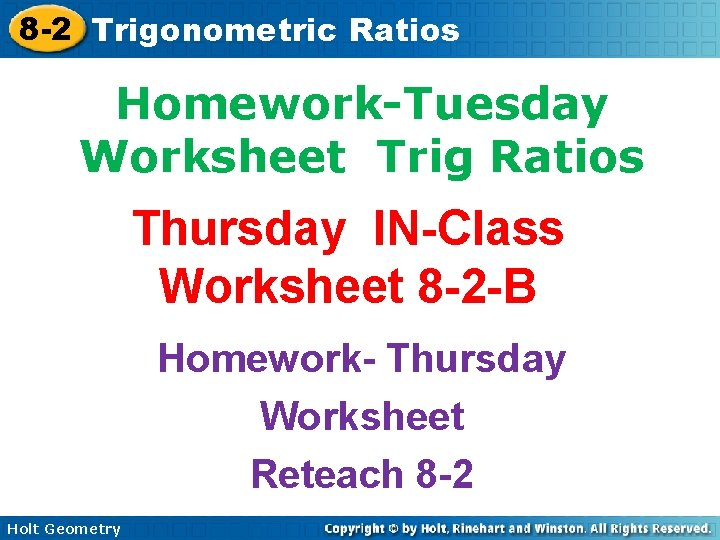8 -2 Trigonometric Ratios Homework-Tuesday Worksheet Trig Ratios Thursday IN-Class Worksheet 8 -2 -B Homework- Thursday Worksheet Reteach 8 -2 Holt Geometry